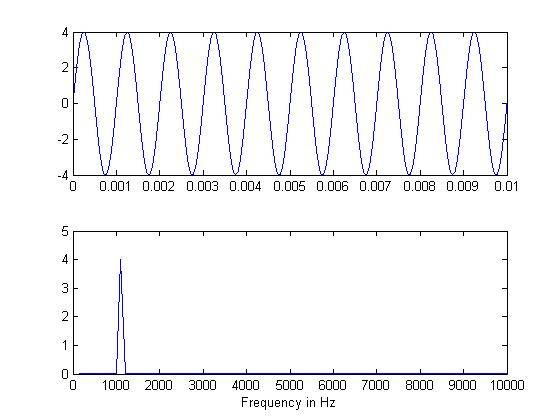Understanding the Image output in Frequency Domain

I need help understanding how frequencies are distributed when taking the FFT of an Image and how can i determine those frequencies, I really need a detailed and easy tutorial with practical example to understand it.

When we take the FFT of a Sine/Cos wave we can easily see its frequencies in FFT, here is my example i added comments in detail to understand,

Code:
%Matlab Code,

f=1e3;   %Frequency of Wave -> 1KHz
A=4;    %Amplitude
Fs = 1e6;  %Sampling Frequency
Ts = 1/Fs;   %Sampling Rate
t = 10/f;       %Time period of 10 Oscillation
n = 0:Ts:t;  %Generating Samples
% (1/f)/T = 1000 %Length for 1 Oscillation after Sampling
% So Length of the Signal for 10 Oscillations is 10,000
x=A*sin(2*pi*f*n);
subplot(2,1 ,1);
plot(n,x);
% 100,000/10,000 = 100Hz <- First point = 100Hz
% 2nd Point = 200Hz
% 3rd Point = 300Hz
% 4th Point = 400Hz
% .
% .
% 10th Point = 1KHz <- Original Signal Frequency
subplot(2,1 ,2);
F=fft(x);
plot(2*abs(F)./(t/Ts));
xlim ([0 100])    % 0 = 100 , 1 = 200......10=1000......100 = 10,000
set(gca, 'XTickLabel', ((get(gca,'XTick')))*100)
xlabel ('Frequency in Hz')

and here is the output image,See how easily I can see the frequency of actual signal in Frequency domain, this is because i knew that the original frequency was 1KHz.

but in case of Images, what are the frequencies ?

here is the simple code,

Code:
image = [0 0 0 0 1 1 1 1]
The above discrete signal has 8 points so N = 8 How can i determine the frequencies and amplitude of this signal ? just like i did above for Sine wave

If i take its FFT and display it ,

Code:
f = fft2(image)
imshow(fftshift(f)); % plottingit is nothing but 3-colors the middle one is white. (as expected)
I've no idea what does that output mean , how could i understand what are the frequencies in the image from this output ?

Can somebody explain me just one example of image FFT and how to determine the frequency etc like i did above in case of Sine wave?

Last edited: